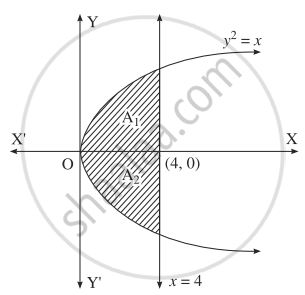# Find the area of the region bounded by the following curves, X-axis and the given lines: y2 = 16x, x = 0, x = 4 - Mathematics and Statistics

Sum

Find the area of the region bounded by the following curves, X-axis and the given lines: y2 = 16x, x = 0, x = 4

#### SolutionThe required area consists of two bounded regions A1 and A2 which are equal in areas.

For y2 = x, y = sqrt(x)

Required area = A1 + A2 = 2A1

= 2int_0^4 y*dx,  "where"  y = sqrt(x)

= 2int_0^4 4 sqrt(x)*dx

= 8[(x^(3/2))/(3/2)]_0^4

= 16/3[x^(3/2)]_0^4

= 16/3[(4)^(3/2) - (0)^(3/2)]

= 16/3 (8)

A = (128)/(3) "sq. units".

Concept: Area Bounded by the Curve, Axis and Line
Is there an error in this question or solution?
Chapter 5: Application of Definite Integration - Exercise 5.1 [Page 187]

#### APPEARS IN

Balbharati Mathematics and Statistics 2 (Arts and Science) 12th Standard HSC Maharashtra State Board
Chapter 5 Application of Definite Integration
Exercise 5.1 | Q 1.7 | Page 187
Share